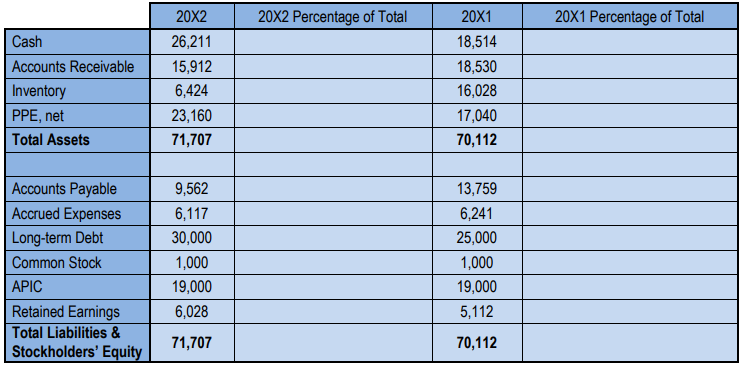Ch. 14 - Financial Statement AnalysisWorksheetSee all chapters
 Ch. 1 - Introduction to Accounting 1hr & 21mins 0% complete Worksheet Ch. 2 - Transaction Analysis 1hr & 14mins 0% complete Worksheet Ch. 3 - Accrual Accounting Concepts 2hrs & 38mins 0% complete Worksheet Ch. 4 - Merchandising Operations 2hrs & 31mins 0% complete Worksheet Ch. 5 - Inventory 1hr & 55mins 0% complete Worksheet Ch. 6 - Internal Controls and Reporting Cash 1hr & 16mins 0% complete Worksheet Ch. 7 - Receivables and Investments 3hrs & 17mins 0% complete Worksheet Ch. 8 - Long Lived Assets 5hrs & 6mins 0% complete Worksheet Ch. 9 - Current Liabilities 2hrs & 19mins 0% complete Worksheet Ch. 10 - Time Value of Money 1hr & 27mins 0% complete Worksheet Ch. 11 - Long Term Liabilities 2hrs & 45mins 0% complete Worksheet Ch. 12 - Stockholders' Equity 2hrs & 15mins 0% complete Worksheet Ch. 13 - Statement of Cash Flows 2hrs & 24mins 0% complete Worksheet Ch. 14 - Financial Statement Analysis 5hrs & 27mins 0% complete Worksheet Ch. 15 - GAAP vs IFRS 56mins 0% complete Worksheet

# Vertical Analysis

See all sections
Sections
Horizontal Analysis
Vertical Analysis
Common-sized Statements
Trend Percentages
Discontinued Operations and Extraordinary Items
Introduction to Ratios
Ratios: Earnings Per Share (EPS)
Ratios: Working Capital and the Current Ratio
Ratios: Quick (Acid Test) Ratio
Ratios: Gross Profit Rate
Ratios: Profit Margin
Ratios: Quality of Earnings Ratio
Ratios: Inventory Turnover
Ratios: Average Days in Inventory
Ratios: Accounts Receivable (AR) Turnover
Ratios: Average Collection Period (Days Sales Outstanding)
Ratios: Return on Assets (ROA)
Ratios: Total Asset Turnover
Ratios: Fixed Asset Turnover
Ratios: Profit Margin x Asset Turnover = Return On Assets
Ratios: Accounts Payable Turnover
Ratios: Days Payable Outstanding (DPO)
Ratios: Times Interest Earned (TIE)
Ratios: Debt to Asset Ratio
Ratios: Debt to Equity Ratio
Ratios: Payout Ratio
Ratios: Dividend Yield Ratio
Ratios: Return on Equity (ROE)
Ratios: DuPont Model for Return on Equity (ROE)
Ratios: Free Cash Flow
Ratios: Price-Earnings Ratio (PE Ratio)
Ratios: Book Value per Share of Common Stock
Ratios: Cash to Monthly Cash Expenses
Ratios: Cash Return on Assets
Ratios: Economic Return from Investing
Ratios: Capital Acquisition Ratio